# Java Program to Reverse Given Number

In this tutorial you will learn about the Java Program to Reverse Given Number and its application with practical example.

## Java Programs to Reverse Given Number

In this tutorial, we will learn to create a Java program that will reverse Given Number using Java programming.

## Prerequisites

Before starting with this tutorial, we assume that you are the best aware of the following Java programming topics:

• Operators in Java Programming.
• Basic Input and Output function in Java Programming.
• Basic Java programming.
• While loop in Java programming.
• Conditional Statements in Java programming.
• Arithmetic operations in Java Programming.

## Program to reverse any number:-

In this program, we will, first, declare the variables for the program. Then we will assign the values to the variables. Now we will use the while loop to reverse the number using the arithmetic expression. At last, we will print the output to the user.

With the help of this program, we can reverse any Number.

## Output:-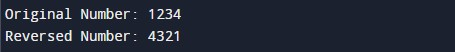In the above program, we have first initialized the required variable.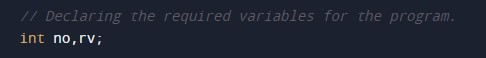• n = it will hold the integer value.
• rv = it will hold the integer value.

Input message for the user for the integer value.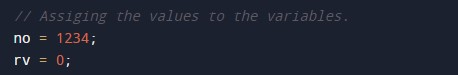Program Logic Code.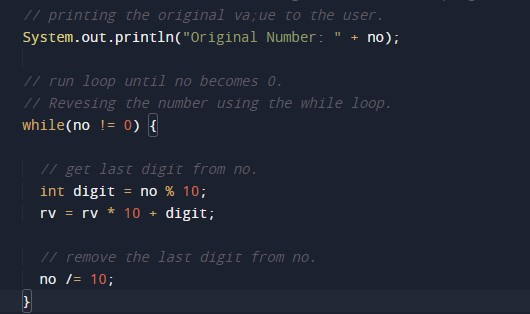Printing output reverses any Number.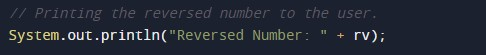In this tutorial we have learn about the Java Program to Reverse Given Number and its application with practical example. I hope you will like this tutorial.